# Catch up on the latest AI articles# Everything You Need To Know About Transformer In Computer Vision! Part5(Video Understanding, Low Shot, Clustering, 3D Analysis)

3 main points
✔️Explain the applications of Transformer in computer vision
✔️Explains research examples in video understanding, low shot, clustering, and 3D analysis tasks

written by Salman Khan, Muzammal Naseer, Munawar Hayat, Syed Waqas Zamir, Fahad Shahbaz Khan, Mubarak Shah
(Submitted on 4 Jan 2021)
Subjects: Computer Vision and Pattern Recognition (cs.CV)

## first of all

Transformer has shown its high performance not only in natural language processing but also in many other areas. Among them, the application research of the Transformer in the field of computer vision, which deals with visual information, has become very popular.

In view of this demand, we will provide a very extensive and detailed description of Transformer in computer vision.

Four models are described for video understanding, two for the low shot task, two for clustering, and three for 3D analysis.

See Parts 2, 3, and 4 for examples of research on other tasks, and Part 1 for a general description of transformers in computer vision.

1. about Transformer in Computer Vision (Part1)

2. A Concrete Example of Transformer in Computer Vision(Part2～5)
2.1 Transformers for Image Recognition(Part2)
2.2 Transformers for Object Detection(Part2)
2.3 Transformers for Segmentation(Part3)
2.4 Transformers for Image Generation(Part3)
2.5 Transformers for Low-level Vision(Part3)
2.7 Video Understanding
・VideoBERT
・PEMT(Parameter Efficient Multi-modal Transformers)
・Video Action Transformer
・Skeleton-based Action Recognition
2.8 Transformers in Low-shot Learning
・CrossTransformers
2.9 Transformers for Clustering
・Set Transformers
2.10 Transformers for 3D Analysis
・Point Transformer
・PCT(Point-cloud Transformer)
・METRO(Mesh Transformer)
3.A Concrete Example of Transformer in Computer Vision(Part1)

## 2.7 Video Understanding

Continuing from Part 4, we present an example of the use of the Transformer for a video understanding task. By nature, some models are strongly related to the multimodal methods introduced in Part 4.

### Video BERT

VideoBERT uses Transformer and self-supervised learning to provide effective multimodal learning for video understanding tasks. The architecture is as follows.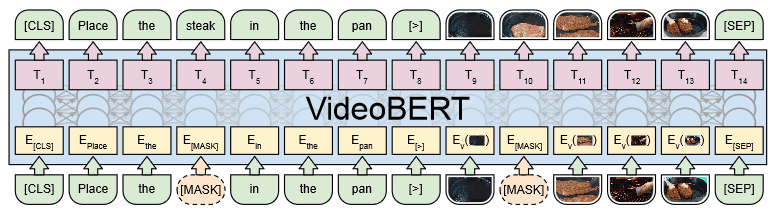As shown in the figure, we use the caption text of the video and the video features as input, and we can see that the structure is very similar to the single stream model of the image-language model introduced in Part 4.

Video features are acquired using a pre-trained S3D model for 30 frames (1.5 seconds) of a 20 fps video. At this time, the video tokens are randomly sub-sampled at a rate of 1 to 5 steps, taking into account the fact that there are differences in the speed of state transitions among videos, even when they correspond to the same action. This makes the system robust to speed variations in the videos and enables us to capture longer term dependencies.

The pre-training tasks are divided into three main types. These correspond to the input data modalities (text only, video only, and text/video) respectively.

Here, for the case where the input data is text only or video only, we train the system to recover the masked tokens as in BERT's MLM.

In contrast, if both text and video are given, we predict whether the text and video match or not. To do this, we use the last hidden state of the [CLS] token. Note, however, that this task is noisy, as the caption text indicates things that are not present in the video.

VideoBERT performed very well on tasks such as action classification, zero-shot classification, and video captioning. Examples of actual caption sentences generated are shown below, where GT is the correct answer sentence and S3D is the caption sentence generated by the existing method.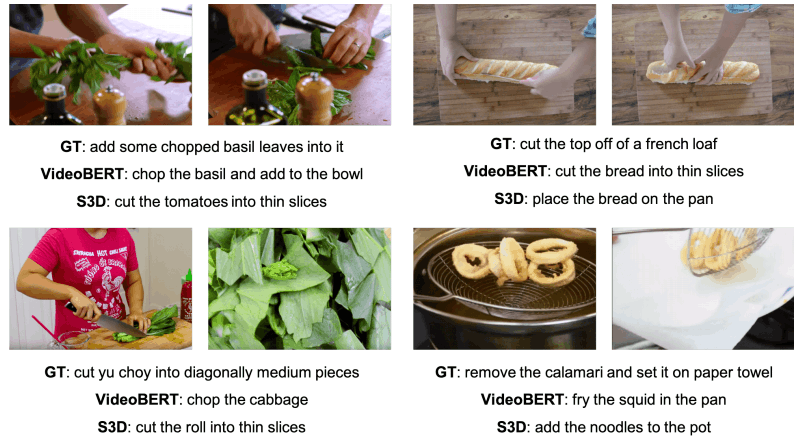### PEMT (Parameter Efficient Multi-modal Transformers)

Existing multimodal methods such as VideoBERT and VilBERT have fixed the language model part as a pre-trained model (e.g. BERT). Therefore, there is a limitation in their ability to learn cross-modal information. In order to solve this problem and realize a multimodal transformer that can be trained end-to-end, the number of parameters needs to be reduced (because it consumes a huge amount of memory).

We have shown that PEMT can train multimodal models (unlabeled visual and audio information in videos) from scratch in an end-to-end manner by reducing the number of parameters by up to 80% through various innovations.

The model architecture is as follows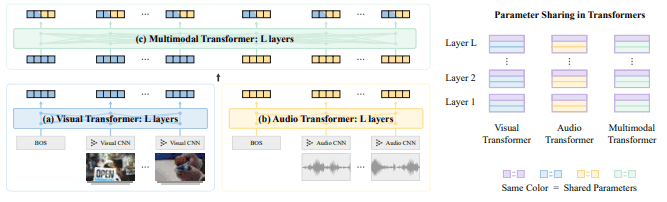The input to this model is a sequence of video clips $v_{1:T}$ with a corresponding sequence of audio streams $a_{1:T}$. These input data are obtained, for example, by dividing a 30-second video into 30 clips (1 second per clip). Based on this diagram, the explanation is shown below.

(1) Local Feature Embedding (corresponding to Visual/Audio CNN in the figure)
These visual and audio data are converted to local feature embedding (Local Feature Embedding) through CNNs respectively. At this time, CNNs are trained not with pre-trained models, but with randomly initialized models in an end-to-end manner.

(2) Unimodal Contextualized Embedding (corresponding to Visual/Audio Transformer in the figure)
As mentioned above, features obtained through CNNs capture only local relationships and cannot capture long-term dependencies. Therefore, visual and audio data are passed through a separate Transformer (Visual/Audio Transformer) to obtain Unimodal Contextualized Embedding that captures long-term dependencies.

(3) Multimodal Contextualized Embedding (corresponding to Multimodal Transformer in the figure)
Finally, to obtain cross-modal information, we use Multimodal Transformer. Through this process, we obtain a contextualized embedding (Multimodal Contextualized Embedding) across the two modalities of visual and audio information.

During the pre-study, you will perform the two tasks listed below.

#### (1) Masked Embedding Prediction (MEP)

Similar to the multimodal model introduced in Part 4, the MLM task is expected to be the first task to learn to recover randomly masked tokens, but since the input data in PEMT is video and audio data, information loss due to discretization of the input is However, in PEMT, the input data is video and audio data.

Therefore, PEMT uses Contrastive Learning to train a model to discriminate between negative samples and correct samples (video clips and audio streams).

Specifically, InfoNCE() is used. At this time, the InfoNCE loss function is as follows.

$L_{NCE}(x,\tilde{o})=-E_x[\sum_tlog\frac{I(x_t,\tilde{o}_t)}{I(x_t,\tilde{o}_t)+\sum_{j∈neg(t)}I(x_j,\tilde{o}_t)}]$

Here, $\tilde{o}_t$ is the $t$th output of any of the three transformers when masking the $t$th input $x_t$. Also, $neg(t)$ is the index of the negative sample, $I(x_t,\tilde{o}_t)=exp(FFN^T(\tilde{O}_t)W_Ix_t)$ (FFN is a two-layer feedforward network).

Also, when sampling negative samples, we prioritize those that are similar to the positive instances in the CNN embedding for effective training.

#### (2)Correct Pair Prediction(CPP)

The MEP task facilitates the learning of the dynamics within each modality. On the other hand, for learning cross-modal dynamics, we use a task that predicts whether video and audio data are derived from the same video (CPP).

The output of the two [BOS], which are the first tokens of the video/audio sequence, or the output of a random position is used to predict the classifier. The loss function is as follows.

$L_{CPP}=-E_{x,y}[c・log p(c|s_g)+c・log p(c|s_h)]$

The final loss function is $L_{MEP}+\alpha L_{CPP}$, with $\alpha=1.0$ for PEMT (the value of $\alpha$ did not have a significant effect on the final performance).

In addition to the weight sharing between the Transformer layers, the model size is greatly reduced by weight sharing between the three Transformers. This is shown in the following figure.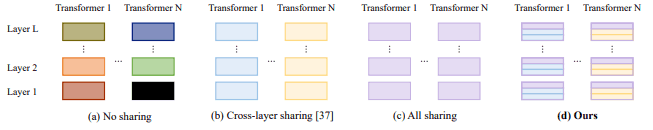In the paper, we have tested different architectures of the multimodal model as introduced in Part 4, such as Early (single stream configuration like VideoBERT and VL-BERT), Mid (two parallel streams configuration like Vil-BERT and LXMERT, identical to the proposed method) and Late (two unimodal Transformer configurations), and late (two unimodal Transformer configurations), as a result of validating different architectures, it is confirmed that the configuration of the aforementioned proposed method is optimal.

Pre-training was performed on the Kinetics-700 dataset, followed by fine-tuning on downstream tasks such as UCF101 (short video classification), ESC (speech classification), and CHarades and Kinetics-Sounds (long-term action recognition). in experiments.

This shows that PEMT can learn valid representationsfrom unlabeled video (video and audio) data for both unimodal tasks related to video and audio respectively, and cross-modal tasks spanning both video and audio.

### Video Action Transformer

Action Transformer is a model for recognizing human actions in a video.

Human action recognition requires information not only about the target person but also about the surrounding people and objects (e.g., "holding an object" or "looking at someone"). Therefore, the goal of this research is to realize a model that can acquire and use contextual imformation of surrounding people and objects related to the target person. The overall flow of the project is as follows.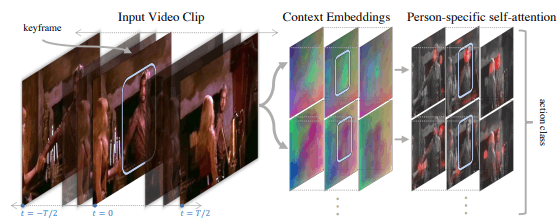In this way, contextual embedding is obtained from the input video, which is then processed by Person-specific self-attention to process information about a specific person and their surroundings for behavior classification.

The architecture is as follows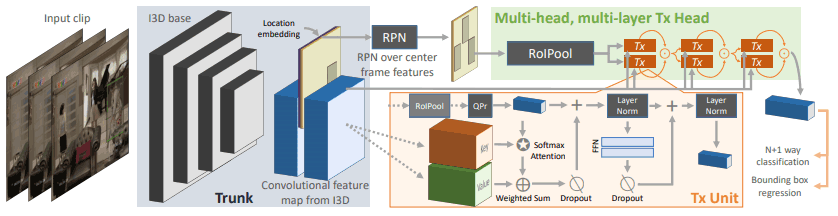First, we use CNN to extract features for 64 frames of clips before and after a given key frame.

The keyframes are then passed through the region proposal network (RPN) to obtain a box and score representing the location of the person present in the keyframe.

Out of these (representing people and their positions in the image), $R(=300)$ boxes with high scores are selected and these are used as query $Q$ for the transformer head. For the key $K$ and value $V$, the part before and after the keyframe is used.

This process allows us to deal with information about a specific person and their surroundings in relation to that person.

The Action Transformer showed excellent performance in the action recognition task. However, it tends to be unsuitable for localization tasks such as identifying the location of a person (because the Transformer tends to incorporate global information rather than local information). Therefore, how to achieve the trade-off between global and local information will be a future challenge.

### ・Skeleton-based Action Recognition

Skeleton-based Action Recognition is a task to perform action recognition based on a human skeleton. In such a task, it is a problem how to obtain and handle not only the relationship between multiple frames of a video but also the relationship between multiple joints.

In this study, Spatial Self-Attention (SSA) and Temporal Self-Attention (TSA) are introduced to handle such temporal and spatial information. The image is shown in the following figure.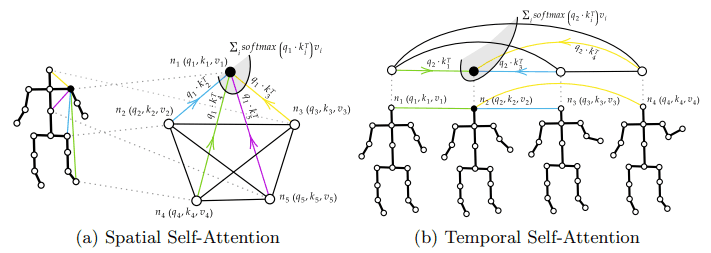Thus, SSA is used to handle (spatial) information between skeletal joints, and TSA is used to handle (temporal) information between multiple frames.

As shown in the figure below, the architecture is a parallel two-stream structure that handles spatial and temporal information respectively.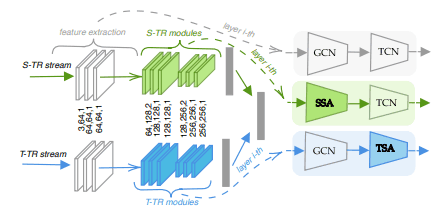where GCN is a graph convolution and TCN is a two-dimensional convolutional layer in the time dimension.

This model (2s-ST-TR) achieves SOTA on the NTU RGB+D 60 and NTU RGB+D 120 data sets.

## 2.8 Transformers in Low-shot Learning

Next, we describe an example application of the Transformer in a Low-shot Learning setting.

### CrossTransformers

CrossTransformers uses self-supervised learning and transformers in the case where the distribution differs between train/test.

The goal here is a low-shot classification task (using Meta-Dataset) where the model is trained on the underlying dataset and then adapted to a new class using a small number of labeled data (the support set).

The paper hypothesizes that the reason for the performance degradation when the distribution changes in the train/test set is that only useful representations of the data in the distribution are retrieved and useful information for the classes outside the distribution is missing, and calls this problem supervision collapse This problem is called supervision collapse. This is illustrated in the following figure.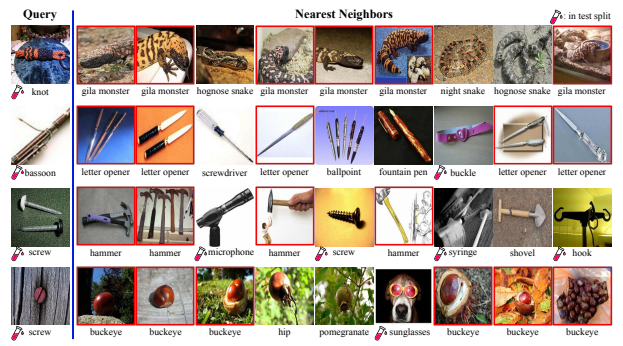For the figure, Query shows the nearest neighbors (up to the top 9) in the train/test support set class for queries retrieved from the Meta-Dataset ImageNet test class, using embeddings learned with Prototypical Net (The test-tube-like marks indicate those in the test set).

Ideally, the nearest neighbors should be in the same class as the query (i.e., data with similar representations as the query are classified in the same class as the query), but in practice, only 5% of them match.

Furthermore, many of the nearest neighbors sample from the same wrong class (red box in the figure), when in fact samples from the same class have very different appearances (most noticeable in the case of buckeye).

This can be interpreted to mean that although we have succeeded in placing representations of the same class of data in close regions in the feature space, we have failed to consider the possibility that there are out-of-distribution samples in the vicinity (resulting in out-of-distribution classes having similar representations to in-distribution classes ).

CrossTransformers applies SimCLR and Transformer to address this problem. The architecture is as follows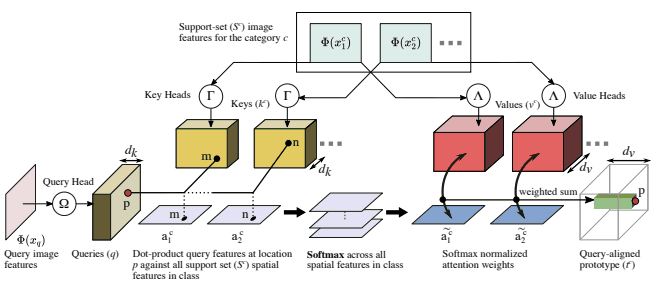Initially, we train a modified version of SimCLR (SimCLR episodes), replacing 50% of the training episodes. This is introduced to avoid the aforementioned embeddings that strongly depend on intra-distributional classes, and to obtain embeddings that are also available for out-of-distributional classes.

Next, we use CrossTransformers as a classifier that uses the embedding thus obtained. This is based on an existing method, Prototypical Nets.

The query $Q$ is obtained from the query image, the key $K$ and value $V$ are obtained from the support set image, and the class decision of the query image is made based on the similar regions and features of the query image and support set image. We omit the details.

This method showed excellent performance in Meta-Dataset, as shown in the following table.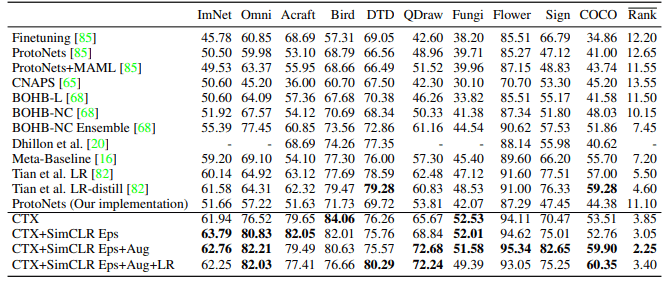FEAT proposes a method that uses set-to-set functions (e.g., Transformer) to adapt the embedding of instances to the target classification task.
The architecture is as follows.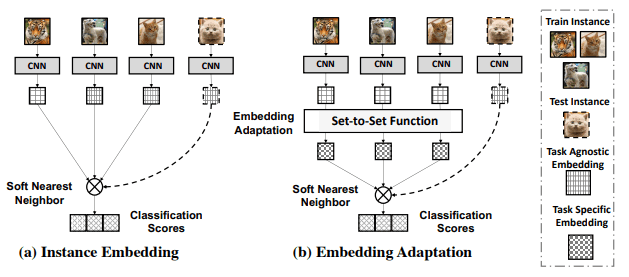In existing methods, it is common to use the same embedding function $E$ for all tasks, but FEAT uses a set-to-set function to obtain task-specific embedding (Figure (b) Embedding Adaptation).

The FEAT model performed well on the inductive/transductive/generalized Few-Shot Learning task.

## 2.9 Set Transformer (Transformers for Clustering)

Clustering is a common unsupervised learning technique that aims to group similar data together.

An example of applying a Transformer to this is the Set Transformer, which addresses a problem called amortized clustering.

Here amortized clustering is a task that learns a parametric function that maps a set of input points to a center in a cluster to which the points belong. Note that this task is characterized by the fact that the output is invariant to the order of the input data.

Set Transformer is aimed at learning such functions by using Transformer.

This Set Transformer is also introduced in the summary on Transformer improvements.

The usual transformer requires $O(N^2)$ of computation for $n$ of input points. To reduce it, ISAB (Induced Set Attention Block) is used as shown in the following figure.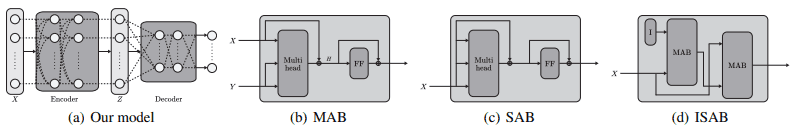(MAB refers to Multi-head Attention Block and SAB refers to Self-Attention Block.)

ISAB makes use of $m$ induced points $I$, with a computational complexity of $O(m・n)$. These guidance points are placed in distributed regions in space and these are compared to the input point set to help solve the task.

In this case, the final model is the Encoder-Decoder model as shown in figure (a). The encoder-decoder is constructed based on SAB or ISAB respectively.

The formulas for Encoder and Decoder are as follows, respectively.

$Encoder(X)=SAB(SAB(X))$ or $Encoder(X)=ISAB_m(ISAB_m(X))$

$Decoder(Z; \lambda)=rFF(SAB(PMA_k(Z))) ∈ R^{k×d}$.

$PMA_k(Z)=MAB(S,rFF(Z)) ∈ R^{k×d}$.

The input $X$ is converted to feature $Z$ by the encoder consisting of SAB or ISAB and converted to output through the decoder.

SetTransformer was evaluated not only for amorized clustering, but also for various set transformation tasks such as counting unique elements in the input set, anomaly detection, and point cloud classification, and showed excellent performance.

There is also emerging research to improve SetTransformer to allow a variable number of clusters by using a sequential method of cluster generation.

## 2.10 Transformers for 3D Analysis

Finally, we present our work on using the Transformer for 3D point cloud analysis. Note that, similar to the amortized clustering described above, the output has the property of being invariant to the order of the input data.

### Point Transformer ( Explanatory article on this site)

Point Transformer is a study of the use of the Transformer for 3D point cloud processing. As mentioned above, the property that the output is invariant to the order of the input set is consistent with the feature of the Transformer that transforms the input set into the output set (natural language processing uses positional encoding to indicate the order of input tokens, but point cloud processing does not need such order information is not necessary, only the coordinates of the points are sufficient).
For more details, please refer to the explanation article in this site.
Point Transformer has shown excellent performance in 3D classification and segmentation tasks.

### PCT(Point-cloud Transformer )

The PCT is a parallel study to the Point Transformer, which is a similar model that uses the Transformer for 3D point cloud processing. However, this one is more similar to the original Transformer architecture.

The architecture is as follows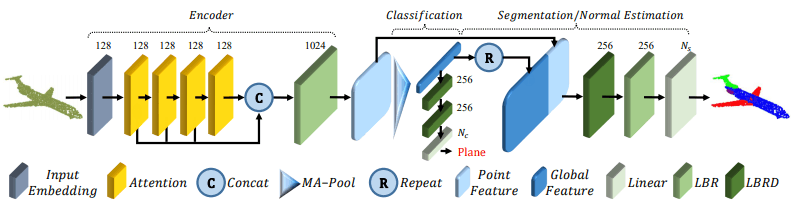I will explain according to this figure. (MA-Pool shows Max pooling and Average pooling, LBR shows Linear layer, BatchNorm and ReLU layer, and LBRD shows LBR with dropout layer added).

• (1) Initially, we are given an input point group $P ∈ R^{N×d}$.
• (2) The input point cloud is transformed into $d_e$-dimensional embedded features $F_e∈R^{N×d_e}$ through the Input Embedding module. (The Input Embedding module is described later in this section.)
• (3)$F_e$ is converted to $F_1,F_2,F_3,F_4$ via four Attention modules. (The Attention module is described later.)
• (4)$F_1,F_2,F_3,F_4$ are concatenated (concat) and converted to features via the LBR layer. At this time, the point-wise feature $F_o=concat(F_1,F_2,F_3,F_4)・W_o$ is later used for the weight $W_o$ of the linear layer of LBR.
• (5) Via Max pooling and Average pooling, $F_g$, a global feature (global feature) that adequately represents the point cloud, is extracted.

Thereafter, it is divided into two major parts depending on the downstream task to be applied.

• For the classification task, the global feature $F_g$ goes through the LBRD layer twice and finally through the linear layer to predict the class.
• For the segmentation task, we concatenate the global feature $F_g$ with the point-wise feature $F_o$. This is followed by point-wise segmentation prediction via LBRD layer, LBR layer and linear layer.

Broadly speaking, it can be summarized as follows.

• (1) Obtaining global and point-wise features with an Attention-based encoder
• (2) For classification task, global features are used.
• (3) For segmentation task, global and point-wise features are used

Here, we will briefly explain the Attention module and Input Embedding module that we omitted earlier.

To begin, as an Attention module, the PCT uses a new method called Offset-Attention. This is shown in the diagram below.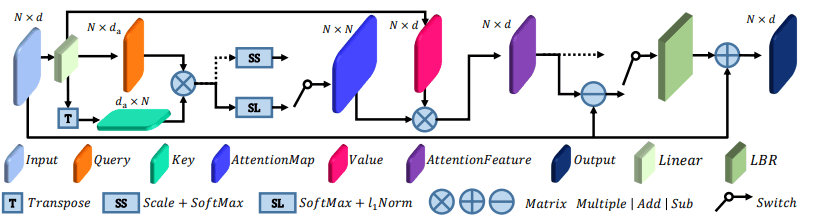It looks complicated, but it is still an Attention process that uses the query $Q$ (orange), the key $K$ (blue-green), and the value $V$ (dark pink).

The important part is the part that calculates the difference (Offset) between the input (Input: light blue) and the feature obtained through the Attention process (AttentionFeature: purple) for each element (the part with the horizontal line in the circle in the figure).

In this case, when the input is $F_{in}$ and the output is $F_out$, the usual Attention is expressed by the following formula.

$F_{out}=LBR(F_{sa})+F_{in} ( F_{sa}=softmax(\frac{(QK^T)V}{\sqrt{d}}) )$

Offset-Attention, on the other hand, looks like this

$F_{out}=LBR(F_{in}-F_{sa})+F_{in}$

It has been found that using Offset-Attention, which is a modification of Attention, resulted in better performance.

Also, the Input Embedding module is as followsThe left side of the figure is called the Neighbor Embedding architecture. It is designed to extract local features successfully.

SG represents the SG module (Sampling and grouping) indicated by the water-dashed line in the center.

Such a process effectively extracts local information-intensive features.

PCT was evaluated on the ModelNet40 and ShapeNet datasets and showed excellent performance on tasks such as 3D shape classification, normal estimation, and segmentation.

### METRO(Mesh Transformer )

The model aims at reconstructing the 3D pose and mesh of a human from a single 2D image. An example of this task is shown below.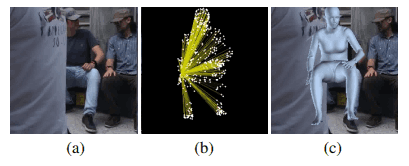In this case, (a) represents the input image, (b) represents the attention between the hand joint and the mesh vertices, and (c) represents the reconstructed mesh.

For this task, it is important to learn the non-local interactions between human joints and mesh vertices (e.g., hands and feet), as illustrated in Figure (b).

To capture these interrelationships in METRO, we use the Transformer as follows.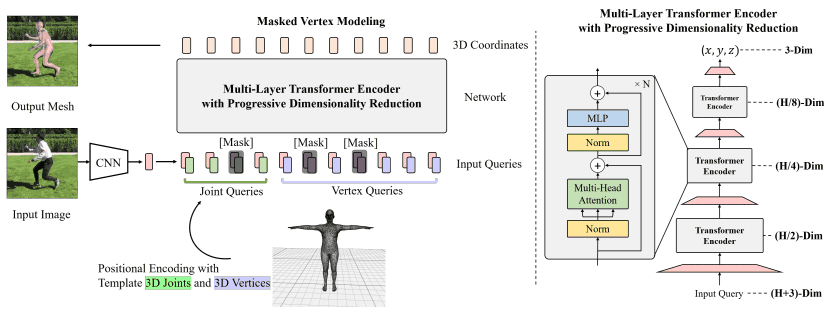A rough description of the model is as follows.

• (1) Given an input image, extract image features using a CNN.
• (2) Add the human mesh template (bottom center of the figure) to the image features. That is, concatenate the 3D coordinates $(x_i,y_i,z_i)$ of each joint $i$ and the 3D coordinates $(x_j,y_j,z_j)$ of each vertex $j$ to the image features.
• (3) Joint Queries and Vertex Queris obtained in (2) are passed through the module represented on the right side of the figure to output 3D coordinates of joints and meshes. This transformer architecture performs sequential dimensionality reduction of features in order to map 2D images to 3D meshes.

During training, Masked Vertex Modeling (MVM) is used to recover masked inputs, similar to BERT's MLM.

METRO achieves SOTA for the 3D human mesh reconstruction task on the Human3.6M, 3D PW dataset. An actual generated example (attention map mesh) is shown below.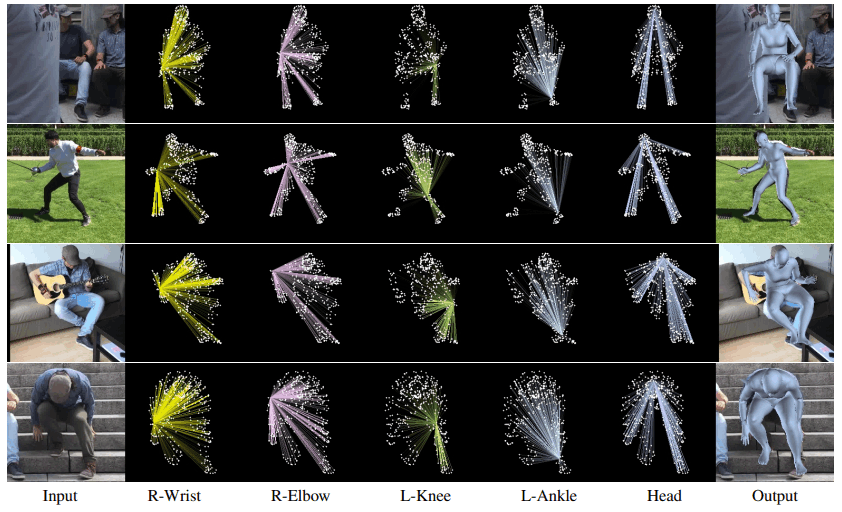In general, we found that Transformer is very capable in 3D point cloud processing.

## summary

In this article, we have provided a detailed description of methods related to video understanding, low shot, clustering, and 3D analysis tasks among transformers in computer vision.

Please refer to Part 2, 3, and 4 for explanations of the methods that could not be introduced in this article, and Part 1 for general explanations.

Transformer has been applied not only to natural language processing, but also to computer vision tasks such as image processing, video processing, and 3D point cloud processing, and has demonstrated excellent performance. Although there are still some issues to be solved with Transformer, research to improve Transformer is in progress, and we will keep an eye on future research.